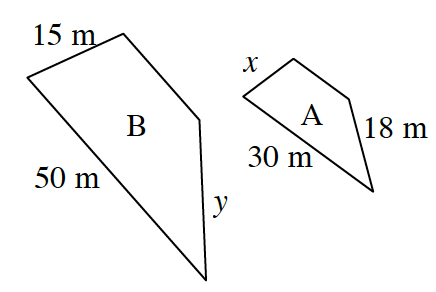### Home > ACC7 > Chapter 7 Unit 8 > Lesson CC3: 7.2.1 > Problem7-40

7-40.The trapezoids at right are similar shapes.

1. What is the scale factor between shape B and shape A?

What is the ratio between the pair of sides 50 m and 30 m?

1. Find the lengths of the missing sides.

Use this ratio with each other pair of sides with one given length to solve for the variable.

$x=$ 9 m

If $x$ is 9 m, what would $y$ be?